Home » Algorithm Time Complexity

# Algorithm Time Complexity

Given a piece of code, how to determine the complexities without much effort? Each piece of code has a  time complexity associated with it which you need to learn. Next time, you see any complex code break it into individual pieces and count the time complexity.

Before you begin, learn the basics of algorithm performance and its complexity.

An algorithm has following types of code and we are going to learn time complexity for each one of them.

1. Statements
2. If-Then-else
3. Loops
4. Nested loops
5. Functions
6. Statements with functions.

## Statements

Each of the statement in an algorithm takes a constant time to execute. If the algorithm procedure has N statements.

Then,

statement 1;

statement 2;

statement 3;

statement n;

Constant time =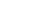Total running time =++ … +.

Sum of all the constant times is also a constant.

Therefore,

The Total running time is also constant.

## If-Then-Else

For the if-then-else block, only one block gets executed, based on the condition. But the run time for the block may be different from other blocks in the if-then-else statement.

if (condition) then
{

block 1;

}
else
{

block2;

}

Therefore,

Total runtime = Max(time(block 1), time(block2));

For Example,

Total runtime =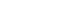,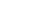.

Depending on the condition the total runtime of if-then-else block could beor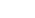.

## Loops

The loop runs for N time for any given N value. The statements inside the loop also get repeated for N times.

for j = 1 to N do {

statements;

}

The loop runs for N time and each of the statements inside the loop takes. Then the total runtime is given below.

Total runtime =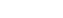=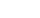.

## Nested Loop

The nested loop is not different than the single loop statement. Now that there are two loops their running time get multiplied.

for i = 1 to N do
{

for j = 1 to M do
{

Statements;

}
}

Suppose the outer loop runs  N times and an inner loop has a complexity of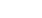.

Total Time Complexity =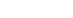Let us say that the inner also runs for N times, the total complexity is given by

=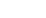.

## Functions

Functions are blocks themselves and contain many other statements. The total time of a function depends on these statements.

Suppose a function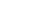takesand there is another function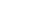that has a loop.

Then,

Total Time Complexity of=because of the loop.

## Statements with Function

A loop statement can be inside of a function, but it is also possible that a function is inside of a loop.

for j=1 to N do {

g(N);

}

Since, we already know that the running time of the functionis.

Total Complexity = N *=This is because the loop runs for N time and repeats the function N times as well.